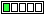All about flooble | fun stuff | Get a free chatterbox | Free JavaScript | Avatarsperplexus dot infoA Quicky III (Posted on 2014-04-09)If each of the a,b,c,d is a decimal digit - for how many ordered quadruplets (a,b,c,d) is the sum S= a+b+6c+d divisible by 3 ?Comments: ( Back to comment list | You must be logged in to post comments.)How to do it quickly Comment 9 of 9 |6c is always divisible by 3. Therefore, a+b+d is divisible by 3, and c can be any digit. Let abd be a 3-digit number from 000 to 999. There are 334 numbers from 000 to 999 that are divisible by 3. Therefore, there are 334 numbers for abd. Since c can be any digit, there are 334*10=3340 quadruplets (a, b, c, d).

 Posted by Math Man on 2014-04-12 22:18:40Please log in:

 Search: Search body:
Forums (0)